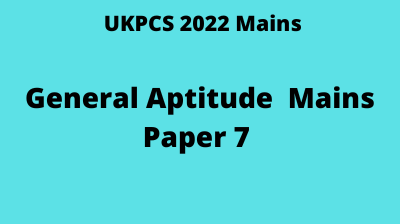# General Aptitude for UKPCS Mains Paper 7 || Oracle IAS

UKPCS Mains 2021 pattern is different from other state civil services exam and UPSC CSE. One of the differentiating factor, the General Aptitude Paper 7. General aptitude for ukpcs

# Oracle IAS analysis and tips for general aptitude for UKPCS:

• Paper 7 consists of General Aptitude and Ethics. The weightage of General Aptitude is 120/200 i.e. 60% of Paper 7.
• General Aptitude could be the most scoring part if prepared well. Aspirants can score as high as 110/120.
• Many students with Math/Engineering  background tend to take this section lightly. Though the questions seem easy at first sight, but since  if an aspirant is out of practice, he/she might not be able to complete the whole paper within time frame.
• Since marks are allotted for each step, aspirants should make it a habit to not apply shortcuts.
• As per our analysis, an aspirant should try to complete the General Aptitude part within 90 min and allocate the remaining time for the Ethics section. General aptitude for ukpsc

## The most important topics as per past years pattern are:

• Percentage
• Profit, Loss and Discount
• Simple Interest and Compound interest
• Ratio and Proportion
• Fractions and Rationalization
• AM, GM & HM
• Time, Speed and Distance
• Work and Time
• Mensuration
• Venn Diagram
• Mean, Median and Mode
• Co-ordinate Geometry

### Mode of delivery:  Online on  Oracle IAS’s LMS

• User id and password will be provided to each candidate.
• Repository of all the questions and answers will be available from Day 1.

### Fees/Cost of the program:

• Complete syllabus coverage with doubt clearing sessions
• Mode: Online on Oracle IAS’s LMS with recorded sessions for revision
• Practice papers
• Total Cost of the Program: Rs. 2500/- Only

Call/WhatsApp 9997453844 for details/enrollment.

### Schedule of the General Aptitude forUKPCS/Class Plan:

 Class No. Date Topic 1 13-Jun Introduction 2 14-Jun Number System 3 15-Jun HCF & LCM, Fraction 4 16-Jun Ratio and Proportion 5 17-Jun Doubt Clearing Session 6 18-Jun Percentage 7 19-Jun Profit and Loss 20-Jun off 8 21-Jun S.I. and C.I. 9 22-Jun S.I. and C.I. 10 23-Jun Time and Work 11 24-Jun Time and Work 12 25-Jun Linear Equations 13 26-Jun Doubt Clearing Session 27-Jun off 14 28-Jun Set Theory 15 29-Jun Set Theory 30-Jun off 16 1-Jul Cartesian System 17 2-Jul Cartesian System 18 3-Jul Cartesian System 4-Jul off 19 5-Jul Triangle, Rectangle, Rhombus, Circe etc. 20 6-Jul Triangle, Rectangle, Rhombus, Circe etc. 21 7-Jul Triangle, Rectangle, Rhombus, Circe etc. 22 8-Jul Doubt Clearing Session 23 9-Jul Statistics 24 10-Jul Statistics 11-Jul off 25 12-Jul Statistics 26 13-Jul Statistics 27 14-Jul Probabilty 28 15-Jul Probabilty 29 16-Jul Probabilty 30 17-Jul Doubt Clearing Session 18-Jul off 31 19-Jul Reasoning 32 20-Jul Reasoning 33 21-Jul Reasoning 34 22-Jul Reasoning

### Syllabus as per the official notification:

• Numbers and their classification: Natural, Real (Rational and Irrational), Integers, Division of
numbers and Prime Numbers, Operations on Real numbers, Power root method for real numbers,
Least Common Multiple (LCM) and Highest Common Factor (HCF) of Integers and their relation & difference.
• Ratio and its Properties, Expressing a given number into a given ratio, Comparison of ratios,
Respective proportion, Proportional relation between two or more numbers.
Exchange of a number into rate of interest and rate of interest into number, Expressing a given
quantity as a percentage of another quantity, conversion of a percentage into decimals and decimals
into percentage, effect of percentage change on any number, two step change of percentage for a
number, percentage excess and percentage shortness, gain percentage, profit and loss percentage,
relation between cost price and selling price.
• Effect of change of principal (P), rate (R) and time (T) on simple interest, repayment of debt in
equal installments, compound interest when the interest is compounded yearly, half-yearly and
quarterly, rate of growth and depreciation, principal ammount, time, rate of interest, difference
between compound and simple interest.
• Problems on basic concepts of work and time.
• Simple linear simultaneous equations.
• Sets, subsets, proper subsets, null set, operations on sets (Union, Intersection, Difference), Venn
diagram.
• Elementary knowledge of triangle, rectangle, square, rhombus and circle, theorems related to their
properties and their parameter & area, volume and surface area of sphere, rectangular and circular
cylinder and cone, cube.
• Cartesian system, plotting of a point and distance formula, section formula, area of a triangle.
Analogies, arrangement, causes and effects, family tree, puzzles based questions, sequences and
series, code based questions on letters of alphabet, syllogism, statement and conclusion, problems
based on clocks.
• Significance, sufficiency, collection, classification of data, frequency, cumulative frequency,
tabulation and presentation of data: simple, multiple, subdivided bar diagram, pie chart, histogram,
frequency curve, frequency polygon, ogives, analysis and interpretation of data.
• Arithmetic mean, geometric mean, harmonic mean, median, mode, combined mean of several sets
of observations, average by increasing and decreasing of elements of sets, quartiles, deciles and
percentiles.

• Classical and statistical definition of probability, addition and multiplication theorems of
probability with simple examples, events, sample space.